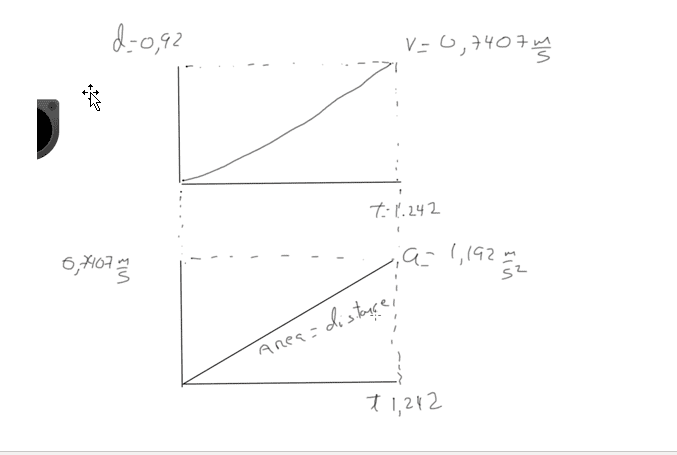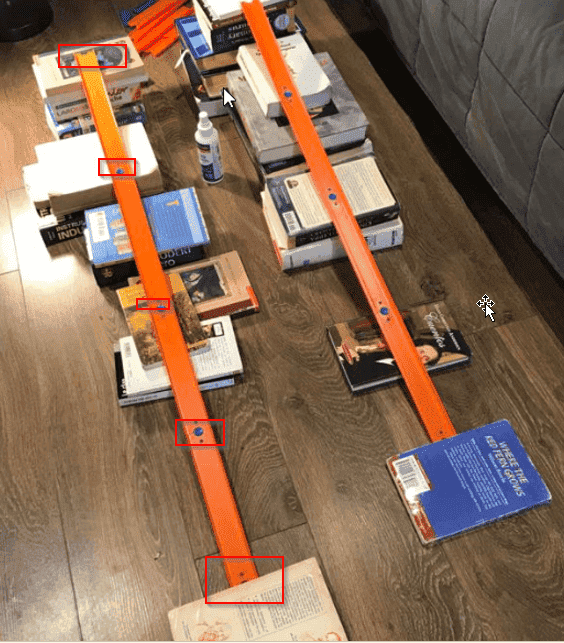# Motion in one dimension -- Experiments with a Hot Wheels car rolling down a ramp

• chucho11028
In summary, the conversation discusses an experiment involving a hot wheels race with a slope and the calculation of velocity using a graph. The question is asked about the error in using the formula for distance d=v*t, where the final velocity is assumed to be increasing constantly and would reflect a parabolic curve. However, it is pointed out that this formula is only valid for constant velocity and with a starting velocity of zero, the final velocity is twice the average velocity. The relevant equation is provided and the mistake is identified as assuming that d/t is the final velocity instead of the average velocity.f

#### chucho11028

Homework Statement
calculate velocity using the graph
Relevant Equations
d=v*t
vf=vo+1/2*a*t
Hello

This is not a homework, this is my own experiment to understand how the motion works. Please, follow my question here below:

I have a hot wheels race with a slope with 10 degrees where I use a small car which departs from the top to the bottom. I have taken 5 times the time to get an average which is 1.242 seconds and the distance is 92 cent which 0.92 m.

Using the formula for distance d=v*t, I know my final velocity is 0.7407 m/s then, I have two graphs:

First graph, velocity and time, where I assume the velocity is increasing constantly and it will reflect a parabolic curve. I have attached the graph

Second graph, velocity and time, where I have a line with a slope which it should be the acceleration.

So, my question is:

I know the area between velocity and time should be the distance, but this area is a triangle, so if I multiply time by velocity and divide by 2 it won’t give me .92 cm. I t will give a half. The only way to get the distance is using the rectangle but it would be wrong. Where is my mistake?

Regards,#### Attachments

Last edited by a moderator:
Homework Statement:: calculate velocity using the graph
Graph of what, precisely ? Is your picture an invented observation, or an actual measurement ?
Relevant Equations:: d=v*t
What are these ? Distance, speed, time ? If yes, the this is only valid if v is constant.
vf=vo+1/2*a*t
What are these ? Final velocity, initial velocity, acceleration, time ? For constant acceleration ?
If yes, then: No, not correct.

I use a small car which departs from the top to the bottom. I have taken 5 times the time to get an average which is 1.242 seconds and the distance is 92 cent which 0.92 m.
So, not 1.244 or 1.240 seconds ? That is really very accurate...It's also very fast. Or is v0 non-zero ?

The graph is not a measure but could you please explain me why the distance in the second graph is only obtained by multiply the area of the rectangle and not the triangle?
v=d/t = 0.92m/ 1.242 sec = 0.7407 m/s and it is totally correct at least there is a new rule in the physics
The acceleration is gives by gravity which is constant
why the graphs just provide the response with 0.92 m if I use the rectangle area instead triangle ?
where is my error or misunderstanding ?

v=d/t ... is totally correct
Any equation can be correct if you define the variables in the right way. How are you defining these? In particular, if d is displacement and t is the time over which the displacement occurs, what, precisely, does v represent?
You seem to be defining it as final velocity, given a zero initial velocity. If so, the equation is wrong.
Maybe you are thinking of ##v_{average}=\frac {\Delta s}{\Delta t}##.

•chucho11028
the v equal to 0.7407 should final velocity, d is displacement and t is the time over which the displacement occurs. The initial velocity should be 0 .
So, the question is, when can I say the area under the graphs (v x t) represents the displacement?
I assume the acceleration is constant because it is the gravity.
Could you please orient me in my misunderstanding?
I would really appreciate

the v equal to 0.7407 should final velocity, d is displacement and t is the time over which the displacement occurs. The initial velocity should be 0

Your error is using " d=v*t " which is valid only for a constant velocity.

Since you indicate yourself that the initial speed is zero, you clearly do not have a constant speed.

Or look in the Homework Help: Introductory Physics Formulary

•chucho11028
my own experiment
I suspect your experimental results consist of a set of five time measurements only. Am I correct ?

In that case you can not draw any graphs and also can not
calculate velocity using the graph

unless you make particular assumptions that you do not mention ...

Last edited:
It's also very fast. Or is v0 non-zero ?
I must withdraw this sceptical and premature remark. Sorry, ignore it.

I know the area between velocity and time should be the distance, but this area is a triangle, so if I multiply time by velocity and divide by 2 it won’t give me .92 cm. I t will give a half. The only way to get the distance is using the rectangle but it would be wrong. Where is my mistake?
Your mistake is assuming that d/t is the final velocity. It is not. It is the average velocity over the time interval t. When the acceleration is constant and the object starts from rest, the final velocity is twice the average velocity. The relevant SUVAT equation is $$d=\frac{1}{2}(v_0+v_f)t=v_{\text{avg.}}t$$ in which ##v_0=0.##

Thus, in your ##v## vs. ##t## drawing the vertical height of the triangle should be ##2\times 0.7407~\text{m/s}=1.481~\text{m/s}.## Then the area under the curve would be ##\text{Area}=\frac{1}{2}\times 1.481~\text{m/s}\times 1.242~\text{s}=0.92~\text{m}## to two significant figures.

•chucho11028
I understood where was my mistake. I assume average velocity as final velocity which is wrong (Big mistake)

I did a resume (attaching here)with my new procedure and possible graph just to leave a possible guide for anyone with similar question

I have a new question based in what I found
I add more segments in my test (I double 1.84) and I curious the average velocity is almost the same 0.77 m/s

in the first test, my acceleration is 1.19.28 m/s^2
the VF=1.4814 m/s

the second test with 1.84 m longitude
acceleration is 0,654061. It is less than before
even is the distance is double, the time is bigger and the acceleration has been reduced (interesting)
but vf= 1.551433 is a little bit high than the vf with 0.92 m
I am not sure the results is because the time that I have taken is not exact or the final velocity is increasing which make sense for me

But my main question is why the acceleration is decreasing here? yeah, it is true the formula says we have double distance divide by time power 2 , but what is the reason the acceleration is decreasing?
I was expecting the acceleration be increasing in order to increase the Vf.
Could you guide me here?

regards,

#### Attachments

• Experimento.docx
247.7 KB · Views: 86
I add more segments in my test (I double 1.84) and I curious the average velocity is almost the same 0.77 m/s
I don't understand what "I add more segments in my test" means. Your parenthetical explanation "I double 1.84" is equally cryptic. What does 1.84 represent? I understand that English is not your primary language, but you need to explain yourself better so that we can understand you. If you recorded data, such as times and distances, please post them and we will help with the analysis. The document that you attached is not much help.

What does 1.84 represent?
Seems to be the length of the ramp:
the second test with 1.84 m longitude
But it looks like the end-to-end height difference is unchanged:
acceleration is 0,654061. It is less than before
the average velocity is almost the same 0.77 m/s

Seems to be the length of the ramp:

But it looks like the end-to-end height difference is unchanged:
Yes, I didn't notice that. This could be a wonderful discovery-type experiment for @chucho11028.

To @chucho11028: Consider this. If the height difference between the ends ##\Delta h## is kept the same, as @haruspex observed, and only the length of the ramp is changed, then the product of the acceleration ##a## and ramp length ##d## is constant, ##a_1d_1 = a_2 d_2.## Your numbers are pretty close to verifying that. Here is an explanation.

A simplified theoretical treatment shows that the acceleration is given by ##a=\dfrac{g\Delta h}{d}##. It is assumed that the object travels distance ##d## and at the same time drops by ##\Delta h.## Also, ##g## = 9.8 m/s2 is the constant acceleration of gravity. You see then that ##ad=g\Delta h## which means that, if you don't change the height difference, the product of acceleration times ramp length is constant; if you increase one of them, the other must decrease to keep the product constant.

This comes from a more detailed treatment which models the small car as a block sliding down the ramp without friction. Dynamical analysis shows that the car's acceleration along the ramp is given by ##a=g\sin\theta## where ##\theta## is the angle of the incline. From trigonometry, ##\sin\theta=\dfrac{\Delta h}{d}## hence the expression for the acceleration that I quoted above.

You might want to test the constancy of the product by varying the length but not the height. Just make sure that the start and end points of the length ##d## traveled by the car on the ramp match the points that you use to measure the height difference ##\Delta h##.